How to use EXACT function in Microsoft Excel

In this article, we will learn how to use EXACT function in Microsoft Excel.

In Excel, EXACT function compares two text strings and return TRUE if they match exactly. Otherwise, it returns FALSE. EXACT is a case-sensitive function, and it takes two arguments –both of which are text strings. It can be a cell reference or typed manually.

Let’s take examples to understand. We have taken 5 different examples.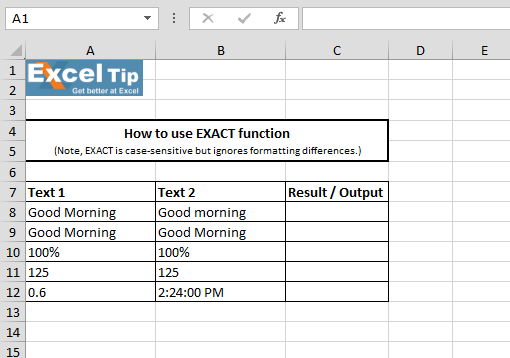1st Example:-

In this example, we will compare the text in two different cells.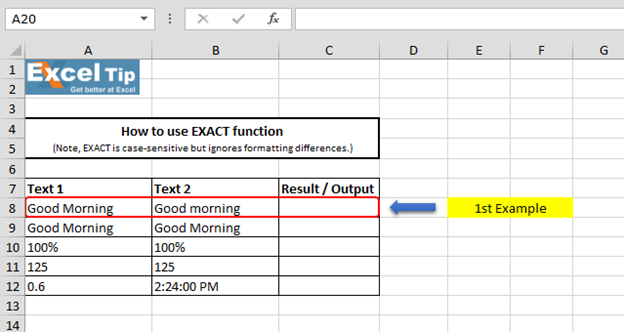• Enter the function in cell C8
• =EXACT(A8,B8)
• Press Enter
• Function returns FALSE, it is because EXACT is case-sensitive function which matches exact text strings. In this example, M of Morning is in upper case in cell A8 and, in cell B8, it is in lower case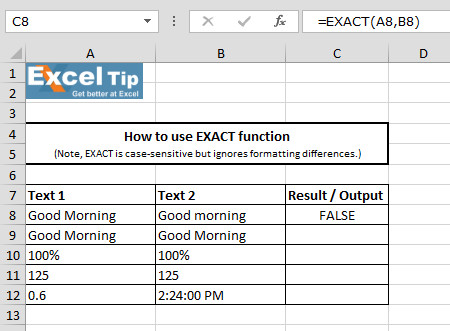2nd Example:-

Compare two cells text.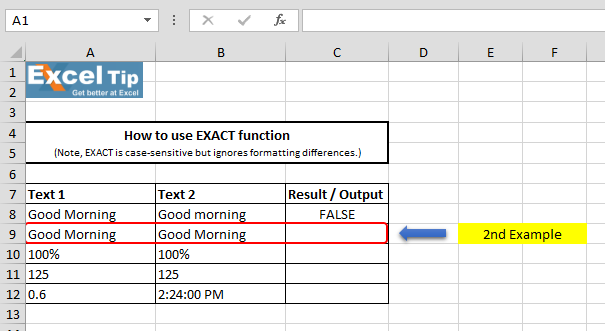• Enter function in cell C9
• =EXACT(A9,B9)
• Press Enter
• Function returns True, because both cell’s text strings are exactly same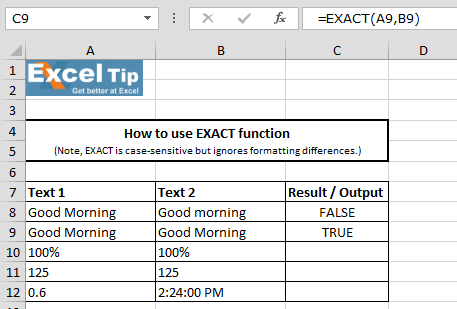3rd Example:-

In this example, we want to compare two different cell’s percentage values.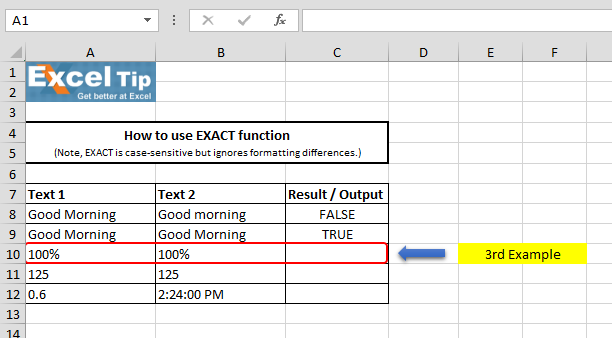• Enter function in cell C10
• =EXACT(A10,B10)
• Press Enter
• The function returns FALSE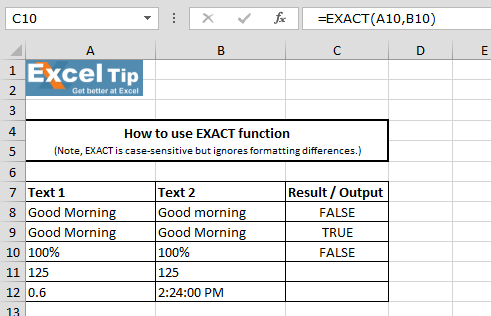It is because cell B10 contains 99.99% and because of cell formatting it is rounding off and displaying 100% that is why function returned FALSE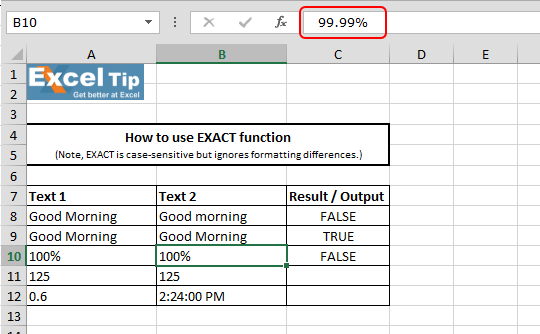4th Example:-

In this example, we’ll match the cell numbers.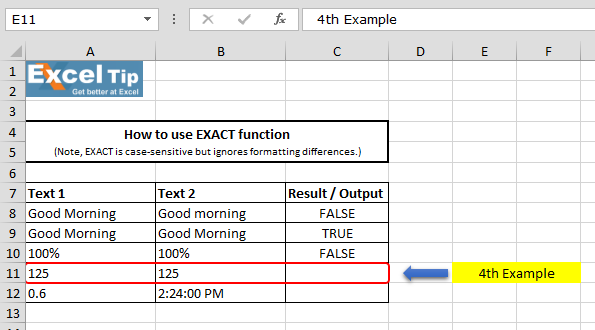• Enter function in cell C11
• =EXACT(A11,B11)
• Press Enter
• The function returns TRUE, because the numbers are matching exactly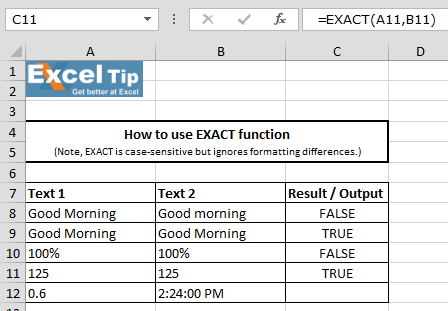5th Example:-

In this example, we’ll match 2 cells value number and time. In the below image, we can see that A12 contains 0.6 whereas B12 has 2:24 PM and it clearly seems that if we enter function, it would definitely return FALSE.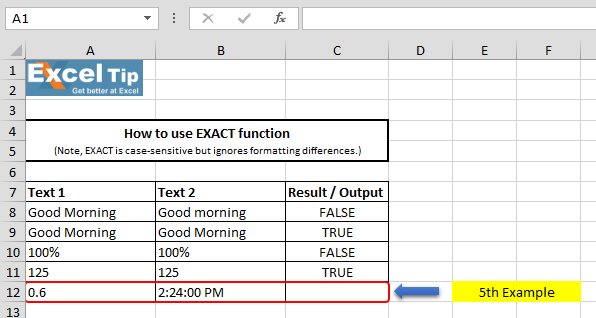• Enter the function in cell C12
• =EXACT(A12,B12)
• Press Enter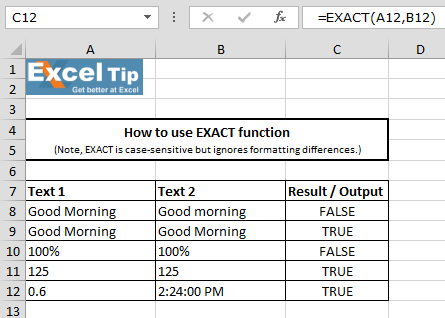The function has given TRUE; it is because the time 2:24 PM in cell B12 actually has the underlying value of 0.6 in Excel. So, if you go to B12 and do right click and go to format cells and under number category, select “General”, then you will see the underlying value of this cell which is 0.6. Therefore, the values in cell A12 & B12 are exactly same and it is just because of formatting that both cells look different.

This is how we can use EXACT function to compare two strings in MS-Excel.# Video: How to use EXACT function in Microsoft Excel

Watch the steps in this short video, and the written instructions are above the video